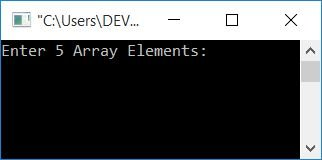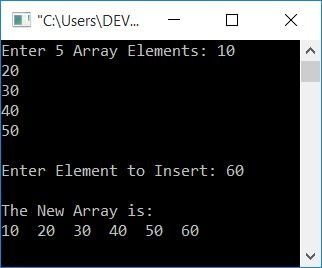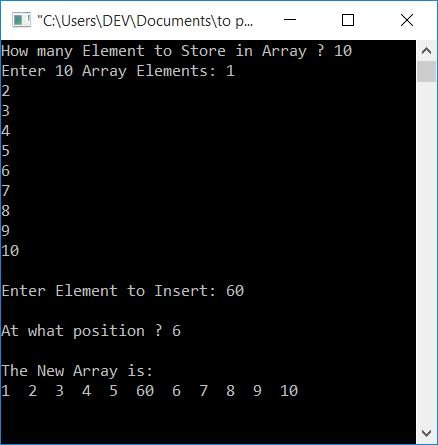# C Program to Insert an Element in an Array

In this article, you will learn and get code about inserting an element in an array in the following ways:

• Inserts an element at the end of an array
• Inserts an Element in a Specific Position

Let's first create a program that asks the user to enter 5 array elements and an element that has to be inserted at the end of the array, as shown in the program given below:

```#include<stdio.h>
#include<conio.h>
int main()
{
int arr, i, element;
printf("Enter 5 Array Elements: ");
for(i=0; i<5; i++)
scanf("%d", &arr[i]);
printf("\nEnter Element to Insert: ");
scanf("%d", &element);
arr[i] = element;
printf("\nThe New Array is:\n");
for(i=0; i<6; i++)
printf("%d  ", arr[i]);
getch();
return 0;
}```

This program was built and run in the Code::Blocks IDE. Here is its sample run:Enter any five numbers as five array elements, say 10, 20, 30, 40, and 50, and then enter an element, say 60, to insert at the end of the array. Here is the sample run:The block of code given below:

```for(i=0; i<5; i++)
scanf("%d", &arr[i]);```

is used to receive the input (as 5 array elements) from the user. When the value of i becomes 5, the condition of the for loop evaluates to false because 5 is not less than 5. So using the statement,

`arr[i] = element;`

The value of the element gets initialized to arr. because i's last value is 5. Therefore, the element gets stored at the last index, or at the end of the given array.

## Insert an Element in a Specific Position

This program inserts an element at a particular position. In this program, the user is allowed to define the size of an array.

```#include<stdio.h>
#include<conio.h>
int main()
{
int arr, i, element, pos, size;
printf("How many Element to Store in Array ? ");
scanf("%d", &size);
printf("Enter %d Array Elements: ", size);
for(i=0; i<size; i++)
scanf("%d", &arr[i]);
printf("\nEnter Element to Insert: ");
scanf("%d", &element);
printf("\nAt what position ? ");
scanf("%d", &pos);
for(i=size; i>=pos; i--)
arr[i] = arr[i-1];
arr[i] = element;
size++;
printf("\nThe New Array is:\n");
for(i=0; i<size; i++)
printf("%d  ", arr[i]);
getch();
return 0;
}```

Let's suppose the user has entered:

• 10 array size
• array elements with the numbers 1, 2, 3, 4, 5, 6, 7, 8, 9, 10
• Insert Element as 60
• 6th position

Then the new array looks like 1, 2, 3, 4, 5, 60, 6, 7, 8, 9, 10. Here is its sample run:As you can see from the sample run given above, elements from the given position (where the new element gets inserted) get shifted one index forward.

Based on the user input given above, the dry run of this program goes like this:

• The entered ten elements (1, 2, 3, ... 10) are set to arr, arr, arr, ... arr.
• Now the value of i is 10.
• 60 (the element to insert) gets initialized to the element variable.
• 6 (position) gets initialized to pos.
• Now shifts all the elements following the given position (6) one index forward, starting from the last element.Therefore,
• arr[i-1] or arr[10-1] or arr or 10 goes to arr[i] or arr.
• The value of i gets decremented and becomes 9. because 9 is greater than the value of pos (6). Therefore again
• arr[i-1] or arr[9-1] or arr or 9 goes to arr[i] or arr.
• and so on, until the value of i becomes less than the value of pos (6).
• In this way, 10, 9, 8, 7, and 6 go to arr , arr , arr , arr , and arr .
• And the current value of i is 5.
• Now, at arr[i] or arr[5,] initialize the element (to insert) 60.
• Because indexing begins at 0, the element at the fifth index is known as the sixth position element.
• Because one extra element gets inserted in the given array. Therefore, increment the size value by 1.
• Finally, print the array.

#### The same program in different languages

C Quiz

« Previous Program Next Program »